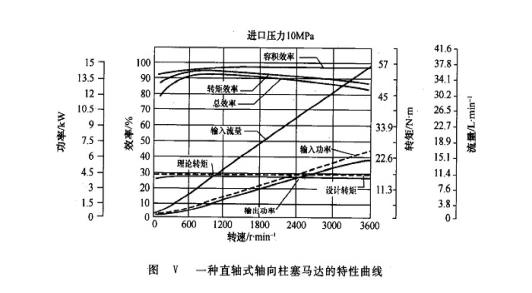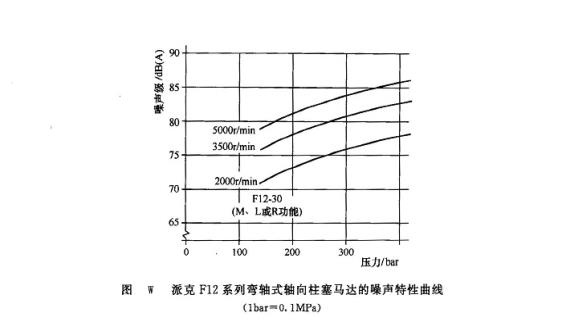Created on：2021-04-09 13:46

# Main performance of axial piston motor

Main performance of axial piston motor

The main performance of axial piston motor includes pressure, displacement, speed, torque, volumetric efficiency and total efficiency.

(1) At present, the maximum pressure of pressure swash plate axial piston motor can reach 40MPa, while the maximum pressure of swash shaft axial piston motor can reach 48mpa; the maximum pressure of double swash plate axial piston motor is slightly lower, up to 32Mpa.

(2) Displacement range and its estimation the displacement estimation method of axial piston motor is the same as that of axial piston pump.

The displacement range of axial piston motor is different from each other: the displacement range of swash plate motor is 2.5 ~ 750ml / R; the displacement range of swash shaft motor is 2.5 ~ 3600ml / R or more; the displacement range of double swash plate motor is also wide, up to 2.5 ~ 3150ml / R.

(3) The speed range of swash plate and swash shaft axial piston motors is wide, the former is 100-3600r / min, the latter is 100-6000r / min. The double swash plate axial piston motor is a low speed motor with a narrow speed range of 10-600r / min.

(4) The instantaneous torque of torque axial piston motor is also pulsating. The average theoretical torque can be calculated as follows:

Tt=V△p/2π=(d2/8)RZ△ptanγ (4-2)

The torque of axial piston motors varies with different types of products, and the starting torque efficiency of all kinds of axial piston motors is basically the same, which is 85% - 95%.

(5) Efficiency the maximum volumetric efficiency of various axial piston motors can basically reach 95%, some even as high as 97%. The highest total efficiency is slightly lower than that of axial piston pump, but it is also as high as 90%. Figure V shows the characteristic curve including efficiency of a straight shaft axial piston motor.(6) The noise level of axial piston motor is higher than that of gear motor, vane motor and screw motor. The noise level of lower swash plate piston motor is the highest, followed by swash shaft motor and double swash plate motor. Figure w shows the noise characteristic curve of Parker F12 series bent shaft axial piston motor.Home    Article    Main performance of axial piston motor